DraftKings Stanley Cup Odds
+450
4.5 to 1Bruins
14.2% implied probability

+600
6 to 1Avalanche
11.2% implied probability

+800
8 to 1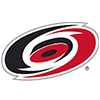Hurricanes
8.7% implied probability

+1000
10 to 1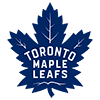Maple Leafs
7.1% implied probability

+1200
12 to 1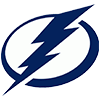Lightning
6.0% implied probability

+1300
13 to 1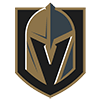Golden Knights
5.6% implied probability

+1400
14 to 1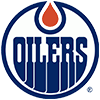Oilers
5.2% implied probability

+1500
15 to 1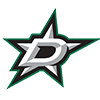Stars
4.9% implied probability

+1600
16 to 1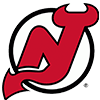Devils
4.6% implied probability

+1600
16 to 1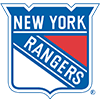Rangers
4.6% implied probability

+2000
20 to 1Wild
3.7% implied probability

+2000
20 to 1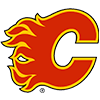Flames
3.7% implied probability

+2500
25 to 1Penguins
3.0% implied probability

+2800
28 to 1Kraken
2.7% implied probability

+2800
28 to 1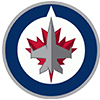Jets
2.7% implied probability

+3500
35 to 1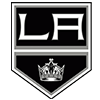Kings
2.2% implied probability

+3500
35 to 1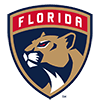Panthers
2.2% implied probability

+4000
40 to 1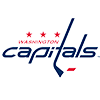Capitals
1.9% implied probability

+6000
60 to 1Predators
1.3% implied probability

+6000
60 to 1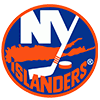Islanders
1.3% implied probability

+10000
100 to 1Sabres
0.8% implied probability

+10000
100 to 1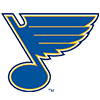Blues
0.8% implied probability

+20000
200 to 1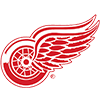Red Wings
0.4% implied probability

+20000
200 to 1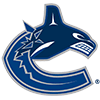Canucks
0.4% implied probability

+20000
200 to 1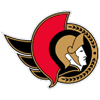Senators
0.4% implied probability

+50000
500 to 1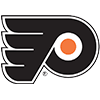Flyers
0.2% implied probability

+100000
1000 to 1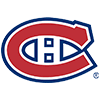Canadiens
0.1% implied probability

+100000
1000 to 1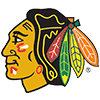Blackhawks
0.1% implied probability

+100000
1000 to 1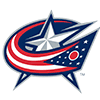Blue Jackets
0.1% implied probability

+100000
1000 to 1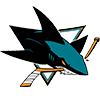Sharks
0.1% implied probability

+100000
1000 to 1Coyotes
0.1% implied probability

+100000
1000 to 1Ducks
0.1% implied probability